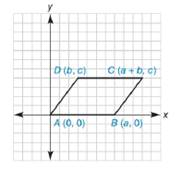Chapter 10.2, Problem 40EElementary Geometry For College St...

7th Edition
Alexander + 2 others
ISBN: 9781337614085

Solutions

Chapter
SectionElementary Geometry For College St...

7th Edition
Alexander + 2 others
ISBN: 9781337614085
Textbook Problem

Show that quadrilateral A B C D is a parallelogram.To determine

To prove:

The quadrilateral ABCD is a parallelogram.Explanation

To prove that the quadrilateral is a parallelogram, opposite sides are need to be parallel.

By theorem,

If two lines are parallel, then their slopes are equal.

(i.e) If l1l2, then m1=m2.

The slope of the line that contains the points x1,y1 and x2,y2 is given by

m=y2-y1x2-x1 for x2x1

Let mAB- and mDC- are the slopes of the line AB- and DC- respectively.

The given points are A0, 0, and Ba, 0.

Using the slope formula and choosing x1=0, x2=a, y1=0, and y2=0.

mAB-=0-0a-0

mAB-=0a

mAB-=0

The given points are Db, c and Ca+b, c.

Using the slope formula and choosing x1=b, x2=a+b, y1=c, and y2=c.

mDC-=c-ca+b-b

mDC-=0a

mDC-=0

Here mAB-=mDC-

Thus, AB DC

Still sussing out bartleby?

Check out a sample textbook solution.

See a sample solution

The Solution to Your Study Problems

Bartleby provides explanations to thousands of textbook problems written by our experts, many with advanced degrees!

Get Started

A population of N = 7 scores has a mean of = 13. What is the value of X for this population?

Essentials of Statistics for The Behavioral Sciences (MindTap Course List)

In Problems 11-22, solve each system by elimination or by any convenient method.

Mathematical Applications for the Management, Life, and Social Sciences

In Exercises 1926, pivot the system about the circled element. [2431|82]

Finite Mathematics for the Managerial, Life, and Social Sciences